Chapter Five Factor Endowments and the HeckscherOhlin Theory

• Slides: 61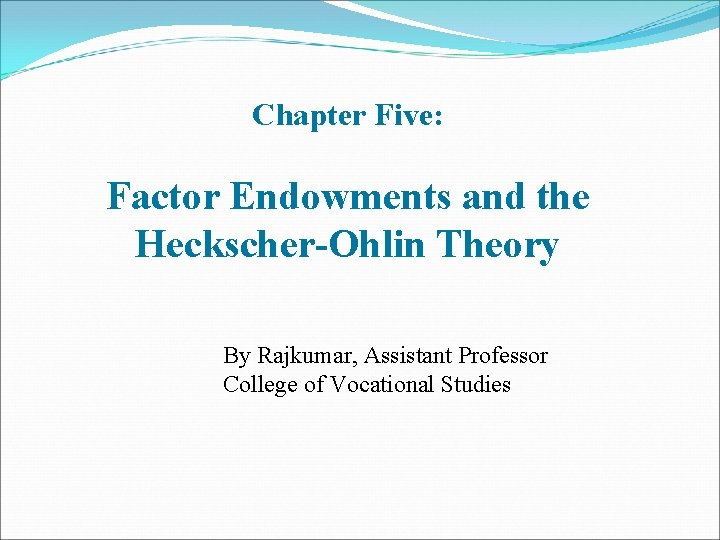Chapter Five: Factor Endowments and the Heckscher-Ohlin Theory By Rajkumar, Assistant Professor College of Vocational Studies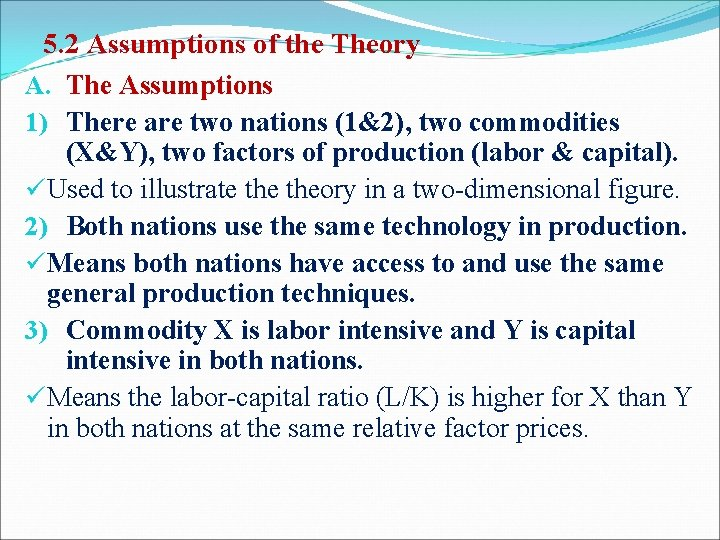5. 2 Assumptions of the Theory A. The Assumptions 1) There are two nations (1&2), two commodities (X&Y), two factors of production (labor & capital). üUsed to illustrate theory in a two-dimensional figure. 2) Both nations use the same technology in production. üMeans both nations have access to and use the same general production techniques. 3) Commodity X is labor intensive and Y is capital intensive in both nations. üMeans the labor-capital ratio (L/K) is higher for X than Y in both nations at the same relative factor prices.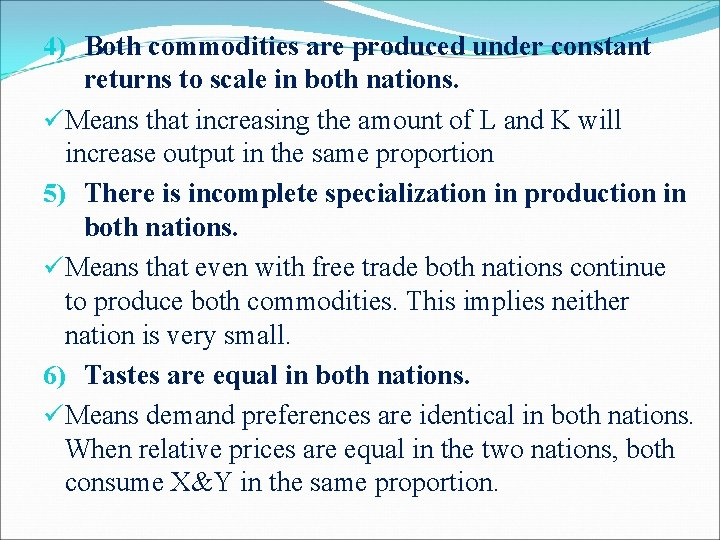4) Both commodities are produced under constant returns to scale in both nations. üMeans that increasing the amount of L and K will increase output in the same proportion 5) There is incomplete specialization in production in both nations. üMeans that even with free trade both nations continue to produce both commodities. This implies neither nation is very small. 6) Tastes are equal in both nations. üMeans demand preferences are identical in both nations. When relative prices are equal in the two nations, both consume X&Y in the same proportion.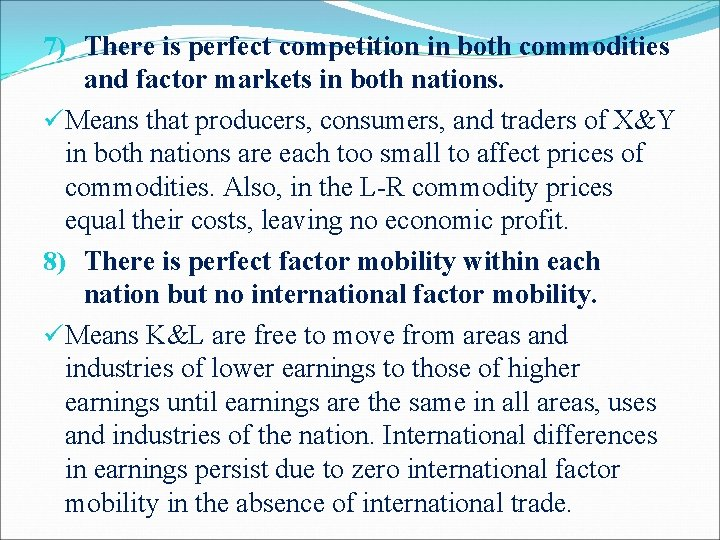7) There is perfect competition in both commodities and factor markets in both nations. üMeans that producers, consumers, and traders of X&Y in both nations are each too small to affect prices of commodities. Also, in the L-R commodity prices equal their costs, leaving no economic profit. 8) There is perfect factor mobility within each nation but no international factor mobility. üMeans K&L are free to move from areas and industries of lower earnings to those of higher earnings until earnings are the same in all areas, uses and industries of the nation. International differences in earnings persist due to zero international factor mobility in the absence of international trade.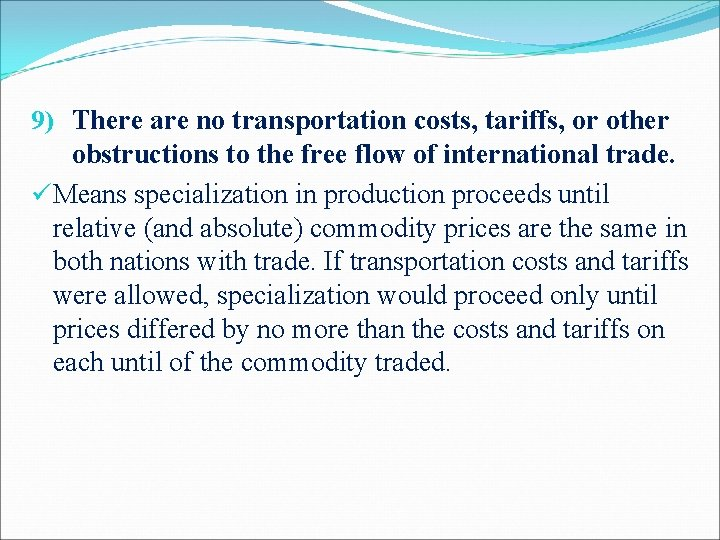9) There are no transportation costs, tariffs, or other obstructions to the free flow of international trade. üMeans specialization in production proceeds until relative (and absolute) commodity prices are the same in both nations with trade. If transportation costs and tariffs were allowed, specialization would proceed only until prices differed by no more than the costs and tariffs on each until of the commodity traded.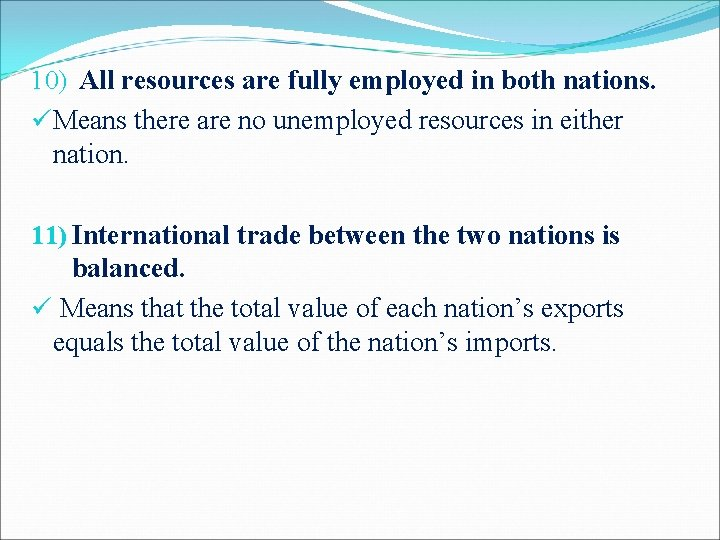10) All resources are fully employed in both nations. üMeans there are no unemployed resources in either nation. 11) International trade between the two nations is balanced. ü Means that the total value of each nation’s exports equals the total value of the nation’s imports.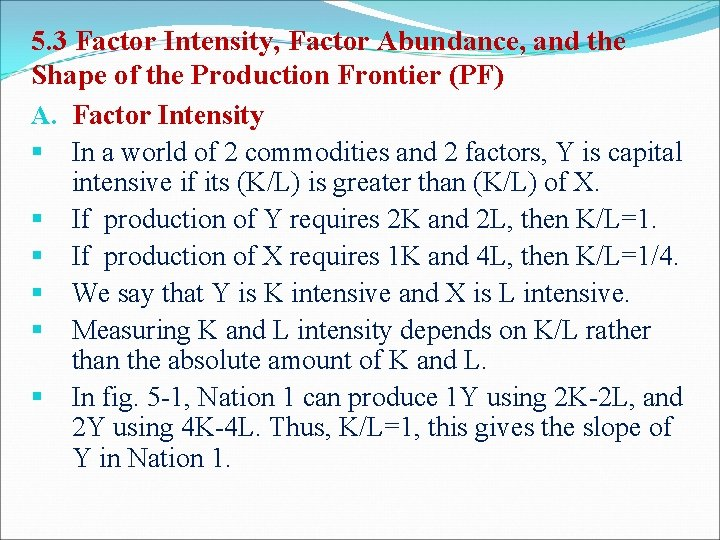5. 3 Factor Intensity, Factor Abundance, and the Shape of the Production Frontier (PF) A. Factor Intensity § In a world of 2 commodities and 2 factors, Y is capital intensive if its (K/L) is greater than (K/L) of X. § If production of Y requires 2 K and 2 L, then K/L=1. § If production of X requires 1 K and 4 L, then K/L=1/4. § We say that Y is K intensive and X is L intensive. § Measuring K and L intensity depends on K/L rather than the absolute amount of K and L. § In fig. 5 -1, Nation 1 can produce 1 Y using 2 K-2 L, and 2 Y using 4 K-4 L. Thus, K/L=1, this gives the slope of Y in Nation 1.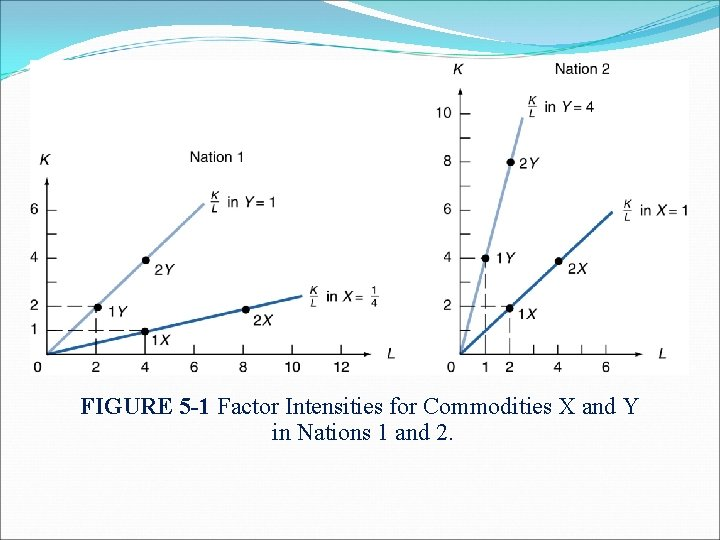FIGURE 5 -1 Factor Intensities for Commodities X and Y in Nations 1 and 2.§ Nation 1 can produce 1 X using 1 K-4 L, and 2 X using 2 K -8 L. Thus, K/L=1/4, this gives the slope of the ray of X in Nation 1. § In Nation 2, K/L=4 for Y and 1 for X. § Therefore, Y is the K-intensive commodity, and X is the L-intensive in Nation 2 also. This is shown by the fact that the ray from the origin for good Y is steeper than that of X in both nations. § Even though Y is K-intensive relative to X in both nations, Nation 2 uses a higher K/L than Nation 1. § For Y, K/L=4 in Nation 2 but K/L=1 in Nation 1. § For X, K/L=1 in Nation 2 but K/L=1/4 in Nation 1.§ Q: Why does Nation 2 use more K-intensive production techniques in both commodities than Nation 1? § A: Capital must be relatively cheaper in Nation 2 than in Nation 1, so that producers in Nation 2 use relatively more capital in the production of both commodities to minimize their costs of production. § Q: But why is capital relatively cheaper in Nation 2? § A: We must define factor abundance and examine its relationship to factor prices. § If the price of capital falls, producers would substitute capital for labor in production of X&Y to minimize production costs. As a result, both commodities become K-intensive. If K/L of Y exceeds K/L of X, Y is considered a K-intensive commodity.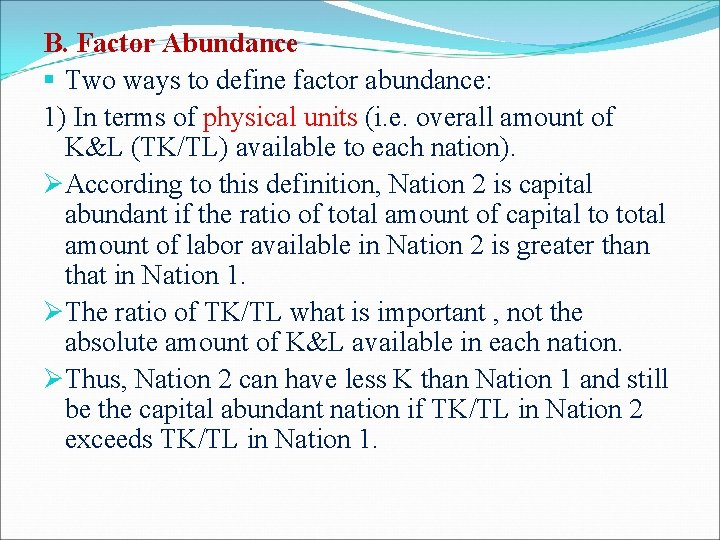B. Factor Abundance § Two ways to define factor abundance: 1) In terms of physical units (i. e. overall amount of K&L (TK/TL) available to each nation). ØAccording to this definition, Nation 2 is capital abundant if the ratio of total amount of capital to total amount of labor available in Nation 2 is greater than that in Nation 1. ØThe ratio of TK/TL what is important , not the absolute amount of K&L available in each nation. ØThus, Nation 2 can have less K than Nation 1 and still be the capital abundant nation if TK/TL in Nation 2 exceeds TK/TL in Nation 1.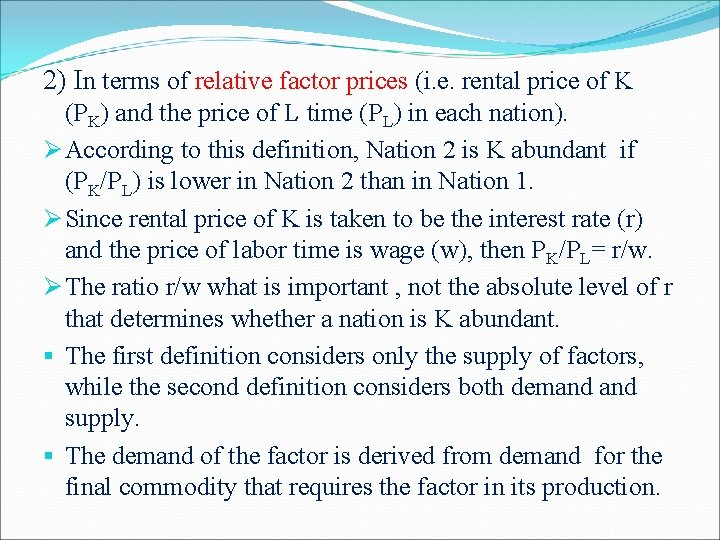2) In terms of relative factor prices (i. e. rental price of K (PK) and the price of L time (PL) in each nation). Ø According to this definition, Nation 2 is K abundant if (PK/PL) is lower in Nation 2 than in Nation 1. Ø Since rental price of K is taken to be the interest rate (r) and the price of labor time is wage (w), then PK/PL= r/w. Ø The ratio r/w what is important , not the absolute level of r that determines whether a nation is K abundant. § The first definition considers only the supply of factors, while the second definition considers both demand supply. § The demand of the factor is derived from demand for the final commodity that requires the factor in its production.C. Factor Abundance and the Shape of the Production Frontier § Since Nation 2 is K-abundant and Y is K-intensive, Nation 2 can produce relatively more of Y than Nation 1. § Since Nation 1 is L-abundant and X is L-intensive, Nation 1 can produce relatively more of X than Nation 2. § This gives a production frontier for Nation 1 that is relatively flatter and wider that of Nation 2.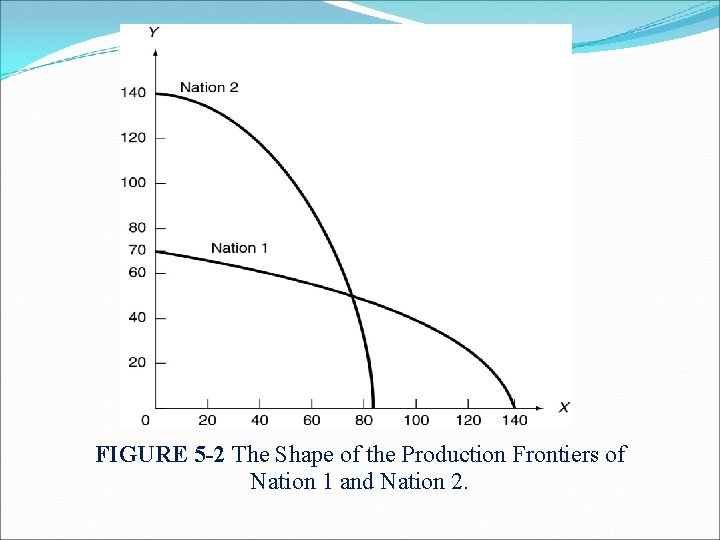FIGURE 5 -2 The Shape of the Production Frontiers of Nation 1 and Nation 2.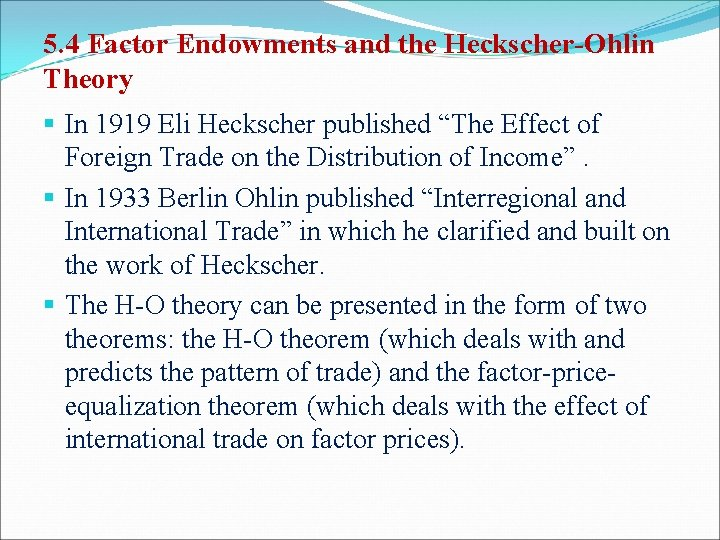5. 4 Factor Endowments and the Heckscher-Ohlin Theory § In 1919 Eli Heckscher published “The Effect of Foreign Trade on the Distribution of Income”. § In 1933 Berlin Ohlin published “Interregional and International Trade” in which he clarified and built on the work of Heckscher. § The H-O theory can be presented in the form of two theorems: the H-O theorem (which deals with and predicts the pattern of trade) and the factor-priceequalization theorem (which deals with the effect of international trade on factor prices).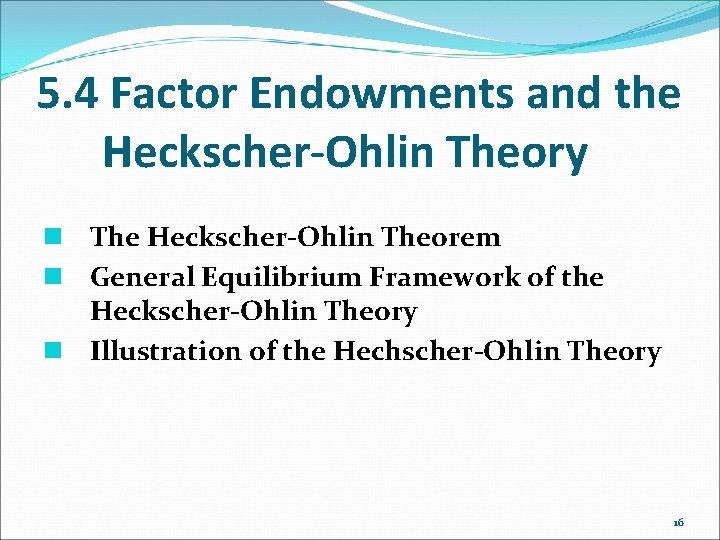5. 4 Factor Endowments and the Heckscher-Ohlin Theory n The Heckscher-Ohlin Theorem n General Equilibrium Framework of the Heckscher-Ohlin Theory n Illustration of the Hechscher-Ohlin Theory 16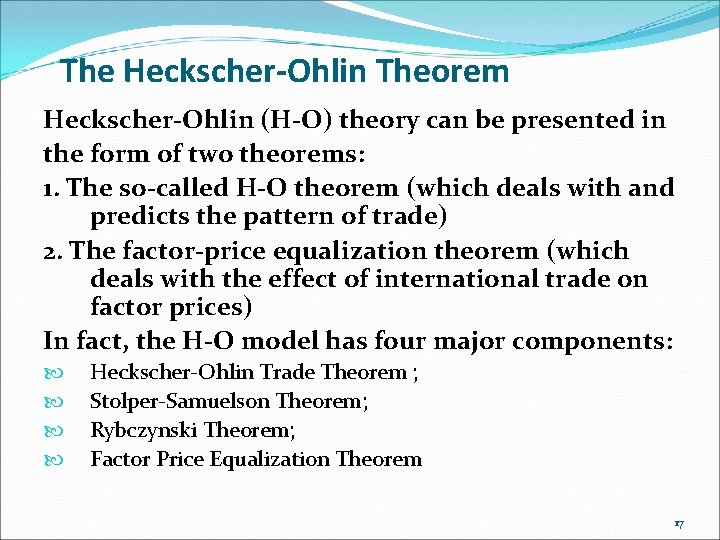The Heckscher-Ohlin Theorem Heckscher-Ohlin (H-O) theory can be presented in the form of two theorems: 1. The so-called H-O theorem (which deals with and predicts the pattern of trade) 2. The factor-price equalization theorem (which deals with the effect of international trade on factor prices) In fact, the H-O model has four major components: Heckscher-Ohlin Trade Theorem ; Stolper-Samuelson Theorem; Rybczynski Theorem; Factor Price Equalization Theorem 17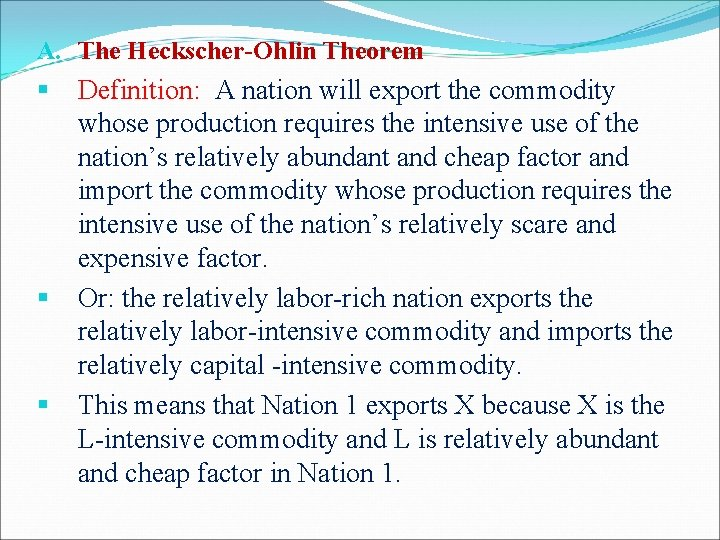A. The Heckscher-Ohlin Theorem § § § Definition: A nation will export the commodity whose production requires the intensive use of the nation’s relatively abundant and cheap factor and import the commodity whose production requires the intensive use of the nation’s relatively scare and expensive factor. Or: the relatively labor-rich nation exports the relatively labor-intensive commodity and imports the relatively capital -intensive commodity. This means that Nation 1 exports X because X is the L-intensive commodity and L is relatively abundant and cheap factor in Nation 1.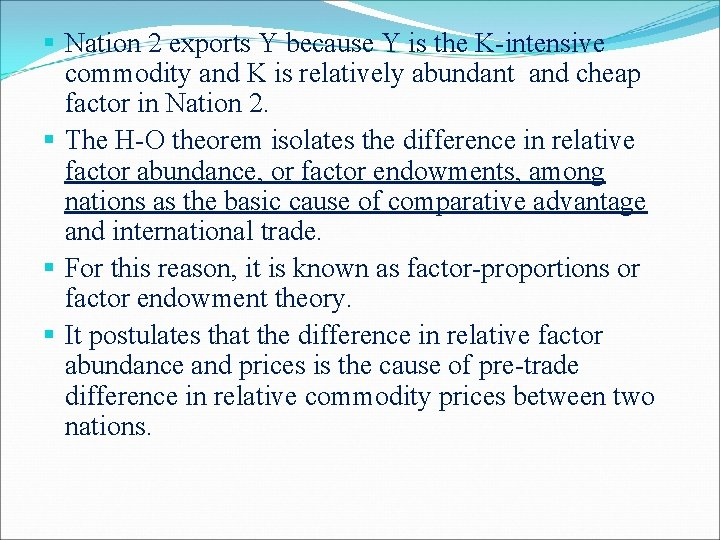§ Nation 2 exports Y because Y is the K-intensive commodity and K is relatively abundant and cheap factor in Nation 2. § The H-O theorem isolates the difference in relative factor abundance, or factor endowments, among nations as the basic cause of comparative advantage and international trade. § For this reason, it is known as factor-proportions or factor endowment theory. § It postulates that the difference in relative factor abundance and prices is the cause of pre-trade difference in relative commodity prices between two nations.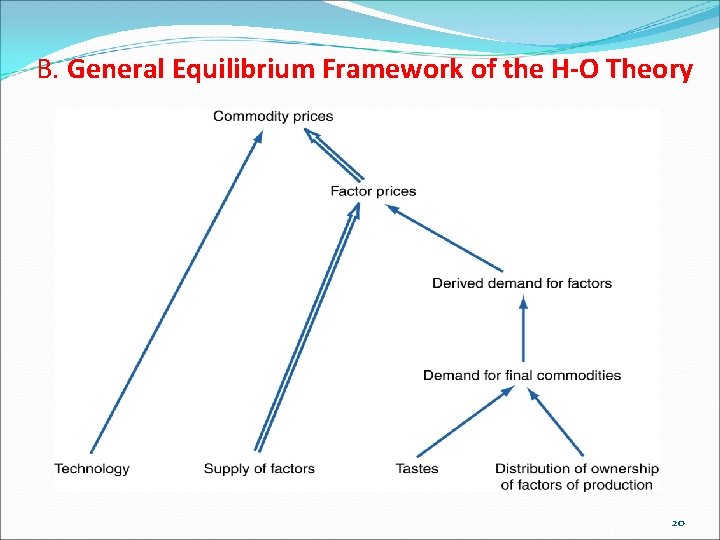B. General Equilibrium Framework of the H-O Theory 20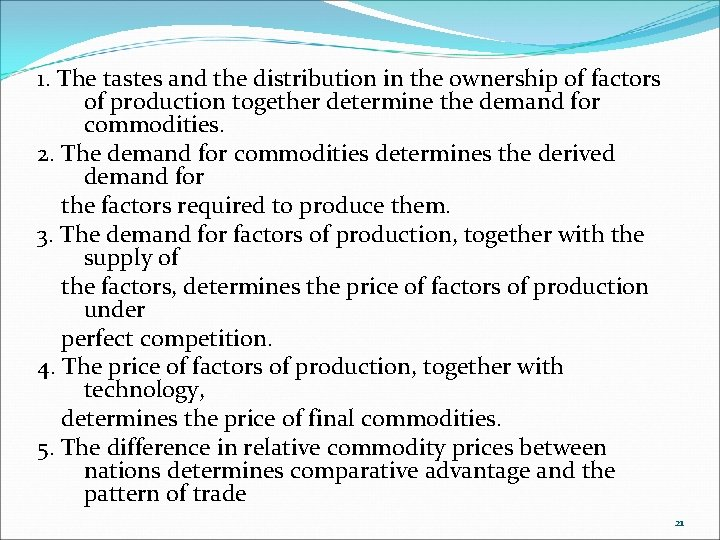1. The tastes and the distribution in the ownership of factors of production together determine the demand for commodities. 2. The demand for commodities determines the derived demand for the factors required to produce them. 3. The demand for factors of production, together with the supply of the factors, determines the price of factors of production under perfect competition. 4. The price of factors of production, together with technology, determines the price of final commodities. 5. The difference in relative commodity prices between nations determines comparative advantage and the pattern of trade 21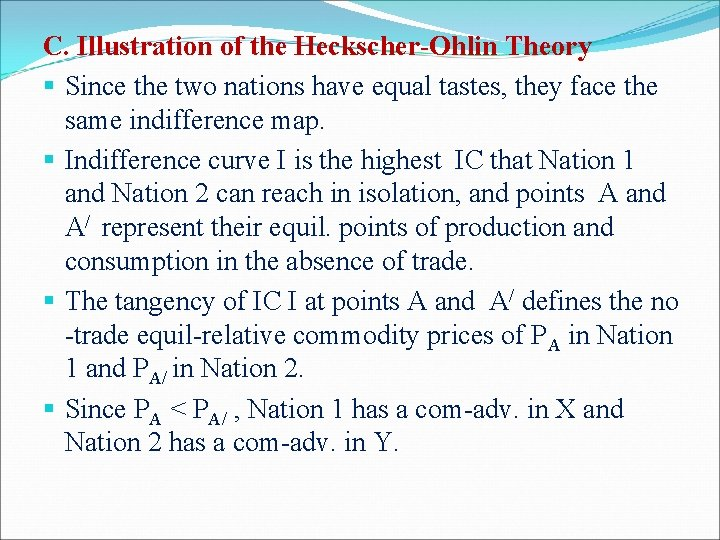C. Illustration of the Heckscher-Ohlin Theory § Since the two nations have equal tastes, they face the same indifference map. § Indifference curve I is the highest IC that Nation 1 and Nation 2 can reach in isolation, and points A and A/ represent their equil. points of production and consumption in the absence of trade. § The tangency of IC I at points A and A/ defines the no -trade equil-relative commodity prices of PA in Nation 1 and PA/ in Nation 2. § Since PA < PA/ , Nation 1 has a com-adv. in X and Nation 2 has a com-adv. in Y.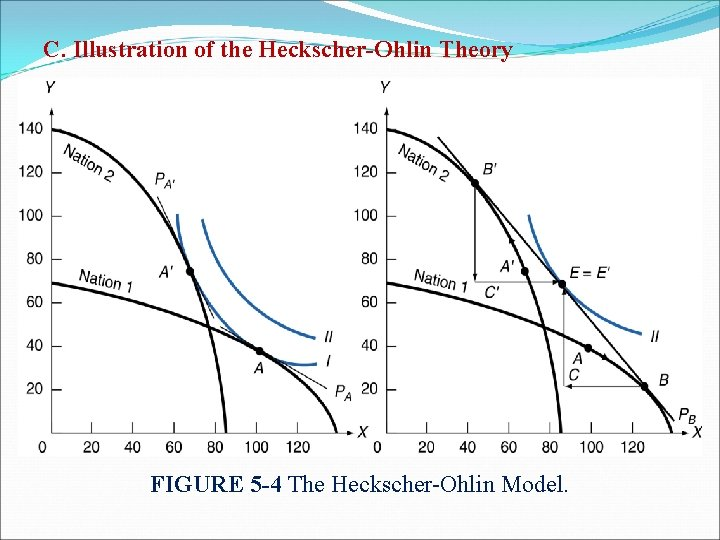C. Illustration of the Heckscher-Ohlin Theory FIGURE 5 -4 The Heckscher-Ohlin Model.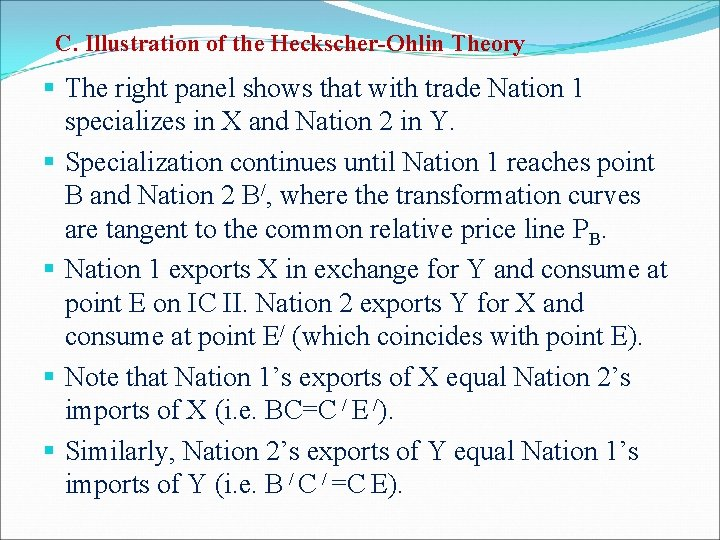C. Illustration of the Heckscher-Ohlin Theory § The right panel shows that with trade Nation 1 specializes in X and Nation 2 in Y. § Specialization continues until Nation 1 reaches point B and Nation 2 B/, where the transformation curves are tangent to the common relative price line PB. § Nation 1 exports X in exchange for Y and consume at point E on IC II. Nation 2 exports Y for X and consume at point E/ (which coincides with point E). § Note that Nation 1’s exports of X equal Nation 2’s imports of X (i. e. BC=C / E /). § Similarly, Nation 2’s exports of Y equal Nation 1’s imports of Y (i. e. B / C / =C E).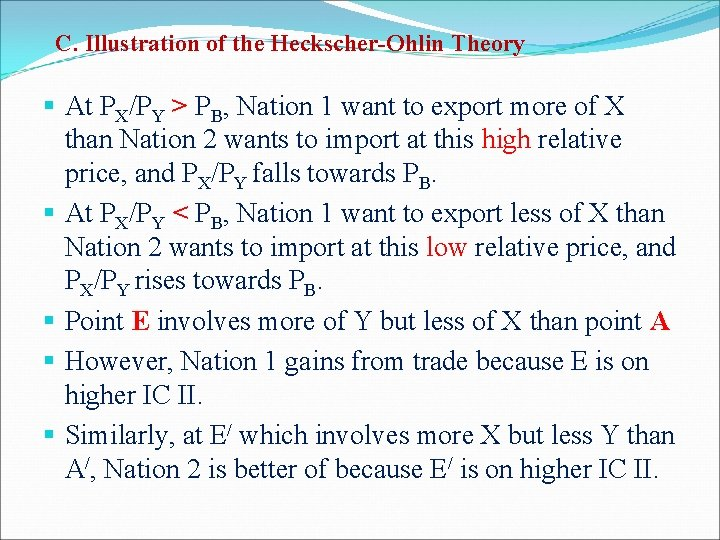C. Illustration of the Heckscher-Ohlin Theory § At PX/PY > PB, Nation 1 want to export more of X than Nation 2 wants to import at this high relative price, and PX/PY falls towards PB. § At PX/PY < PB, Nation 1 want to export less of X than Nation 2 wants to import at this low relative price, and PX/PY rises towards PB. § Point E involves more of Y but less of X than point A § However, Nation 1 gains from trade because E is on higher IC II. § Similarly, at E/ which involves more X but less Y than A/, Nation 2 is better of because E/ is on higher IC II.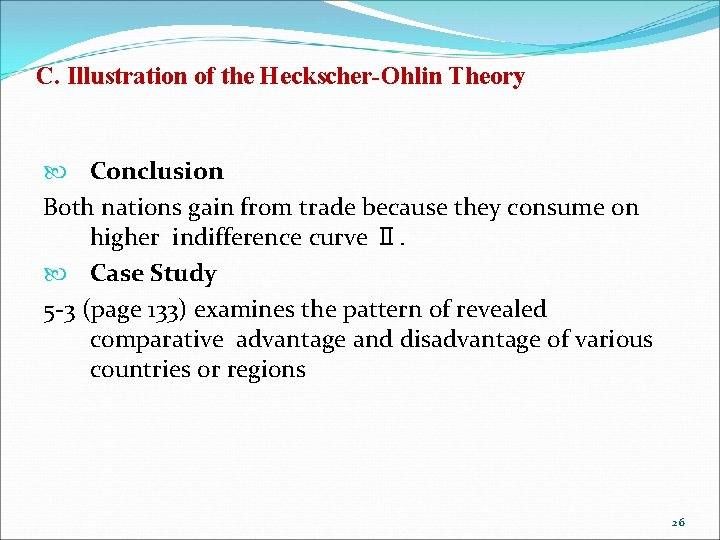C. Illustration of the Heckscher-Ohlin Theory Conclusion Both nations gain from trade because they consume on higher indifference curve Ⅱ. Case Study 5 -3 (page 133) examines the pattern of revealed comparative advantage and disadvantage of various countries or regions 26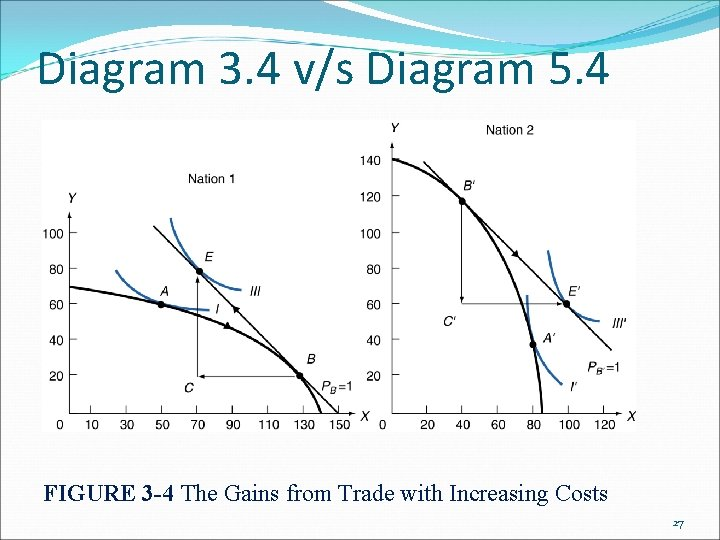Diagram 3. 4 v/s Diagram 5. 4 FIGURE 3 -4 The Gains from Trade with Increasing Costs 27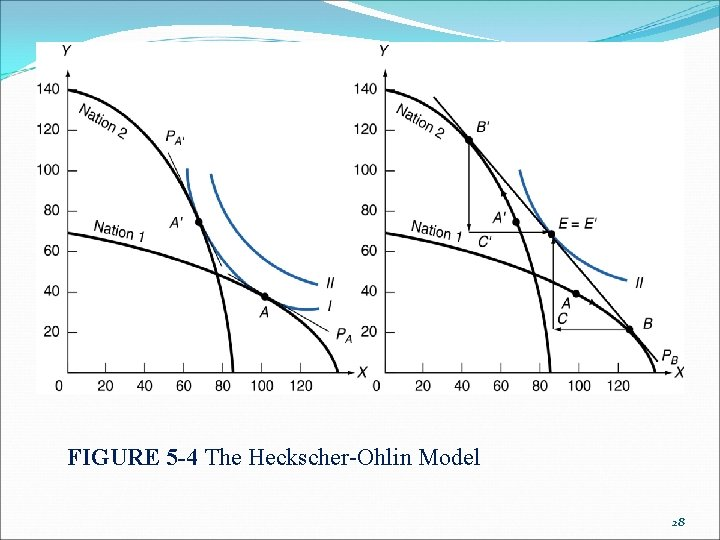FIGURE 5 -4 The Heckscher-Ohlin Model 28Differences In Fig 3. 4, the difference in the production frontiers of the two nations is reinforced by their difference in tastes, thus making the autarky relative commodity price in the two nation differ even more than in Fig 5. 4. 29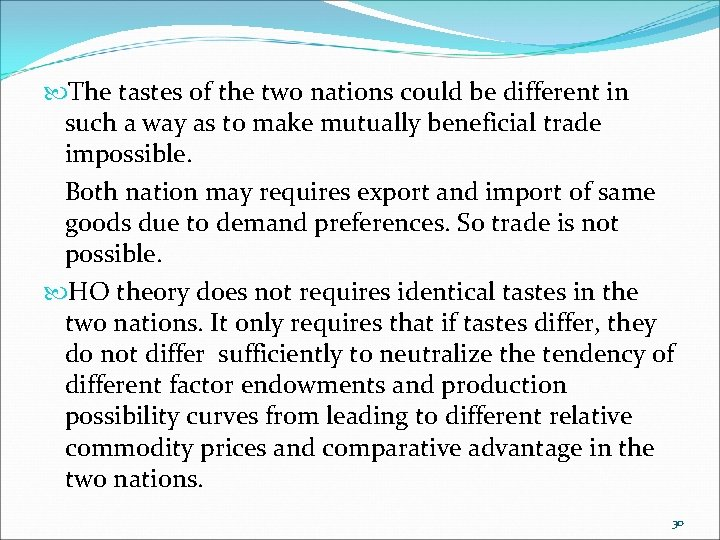The tastes of the two nations could be different in such a way as to make mutually beneficial trade impossible. Both nation may requires export and import of same goods due to demand preferences. So trade is not possible. HO theory does not requires identical tastes in the two nations. It only requires that if tastes differ, they do not differ sufficiently to neutralize the tendency of different factor endowments and production possibility curves from leading to different relative commodity prices and comparative advantage in the two nations. 30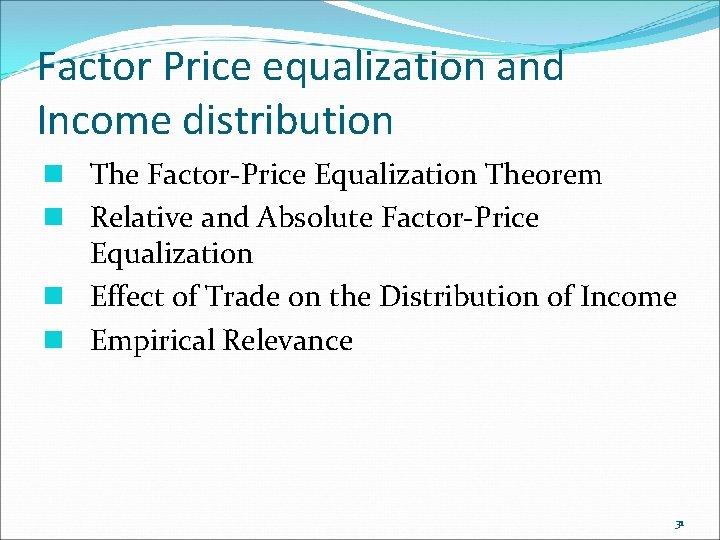Factor Price equalization and Income distribution n The Factor-Price Equalization Theorem n Relative and Absolute Factor-Price Equalization n Effect of Trade on the Distribution of Income n Empirical Relevance 31Additional Topics Stolper-Samuelson Theorem; Rybczynski Theorem; 32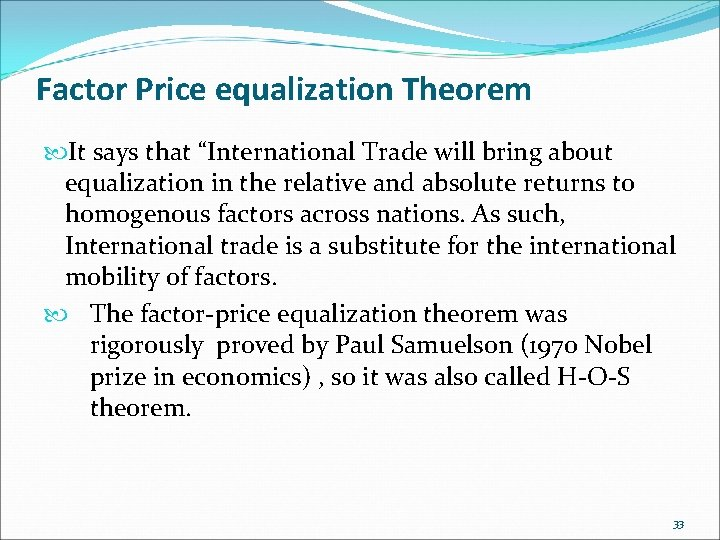Factor Price equalization Theorem It says that “International Trade will bring about equalization in the relative and absolute returns to homogenous factors across nations. As such, International trade is a substitute for the international mobility of factors. The factor-price equalization theorem was rigorously proved by Paul Samuelson (1970 Nobel prize in economics) , so it was also called H-O-S theorem. 33Factor Price equalization Theorem Production Adjustment due to International Trade As the production of the good using the abundant resource intensively increases, demand for that resource will increase; so will the demand for the scarce resource, but by a smaller amount As the production of the good that uses the scarce resource intensively decreases, both abundant and scarce resources will be released, but relatively more of the scarce resource will be released than the abundant resource We can show this production adjustment in following fig. 34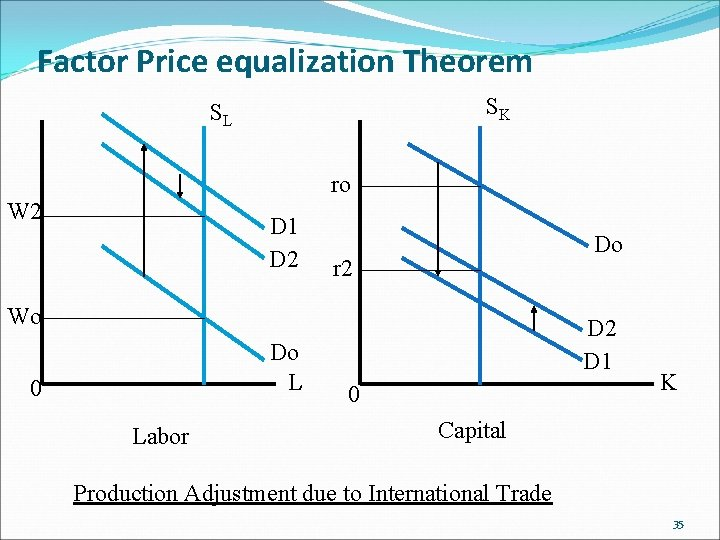Factor Price equalization Theorem SK SL ro W 2 D 1 D 2 Do r 2 Wo Do L 0 Labor D 2 D 1 0 K Capital Production Adjustment due to International Trade 35Factor Price equalization Theorem Explanation of H-O-S theorem 1. In Nation 1 the relative price of commodity X is lower than in Nation 2, it means that the relative price of labor or wage rate is lower in Nation 1 in the absence of trade; 2. With trade, Nation 1 specializes in the production of commodity X (L-intensive commodity) and reduces its production of commodity Y(K-intensive commodity), the demand for labor rises causes the wages to rise while the relative demand for capital falls and its rate falls; on the other hand, in Nation 2 wages fall and rate rises; 36Factor Price equalization Theorem Conclusion 1. International trade tends to reduce the pre-trade difference in w and r between the two nations; 2. International trade keeps expanding until relative commodity prices are completely equalized, which means that relative factor prices have also become equal in two nations. 37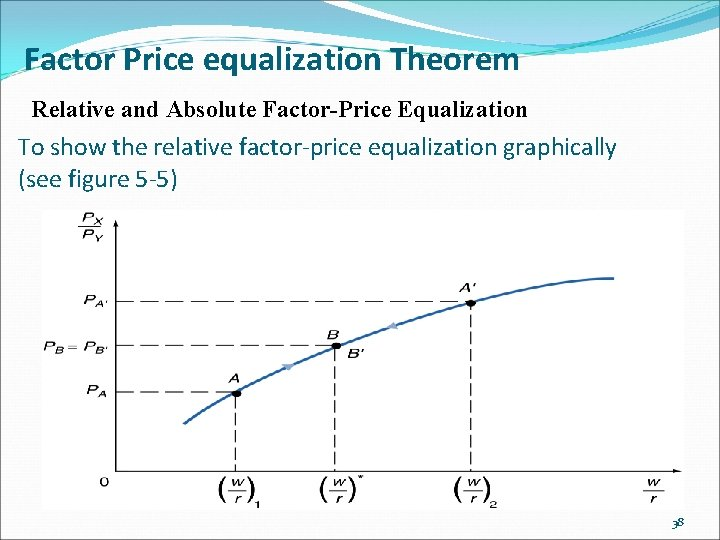Factor Price equalization Theorem Relative and Absolute Factor-Price Equalization To show the relative factor-price equalization graphically (see figure 5 -5) 38Factor Price equalization Theorem Relative and Absolute Factor-Price Equalization u To explain Figure 5 -5 1. The horizontal axis measures the relative price of labor (w/r) while the vertical axis measures the relative price of commodity X (PX/PY); 2. Each w/r is associated with a specific PX/PY ratio (due to the perfect competition and uses the same technology, one to one relationship between w/r and PX/PY); 3. Without trade, Nation 1 is at Point A with w/r=(w/r)1 and PX/PY=PA while Nation 2 is at Point A’ with w/r=(w/r)2 and PX/PY=PA’; 39Factor Price equalization Theorem Relative and Absolute Factor-Price Equalization 4. With trade, Nation 1 will produce more of commodity X due to the PA � PA’ in the relative price of commodity X in Nation 1 than Nation 2 while Nation 2 will produce more of commodity Y. 5. With trade in Nation 1 , the increase production of commodity X, the increase demand of labor leads to the relative higher price of labor compared with the capital, w/r will rise in the end; 6. With trade in Nation 2 , the increase production of commodity Y, the increase demand of capital leads to the relative higher price of capital compared with the labor, r/w will rise (w/r will fall) in the end; 40Factor Price equalization Theorem Relative and Absolute Factor-Price Equalization 7. The upward movement in Nation 1 and downward movement in Nation 2 will continue until point B=B’, at which PB=PB’ and w/r=(w/r) � (only at this point both nations operate under perfection competition and use the same technology by assumption) u To summarize PX/PY will become equal as a result of trade, and this will only occur when w/r has also become equal in the two nations (as long as both nations continue to produce both commodities). 41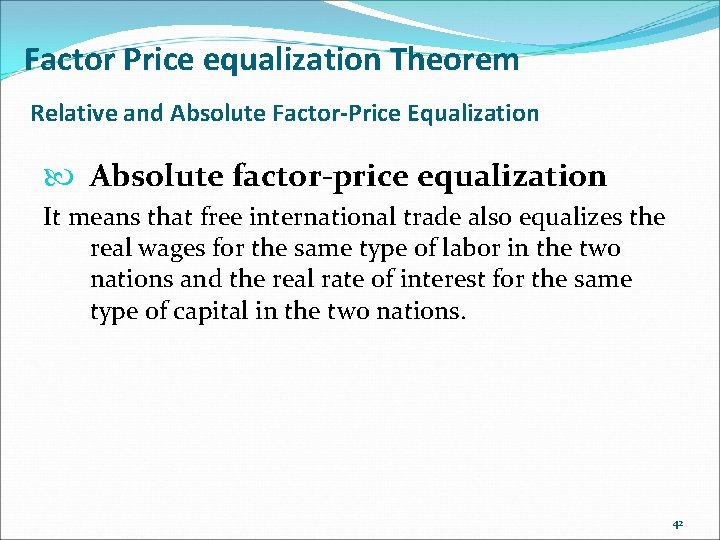Factor Price equalization Theorem Relative and Absolute Factor-Price Equalization Absolute factor-price equalization It means that free international trade also equalizes the real wages for the same type of labor in the two nations and the real rate of interest for the same type of capital in the two nations. 42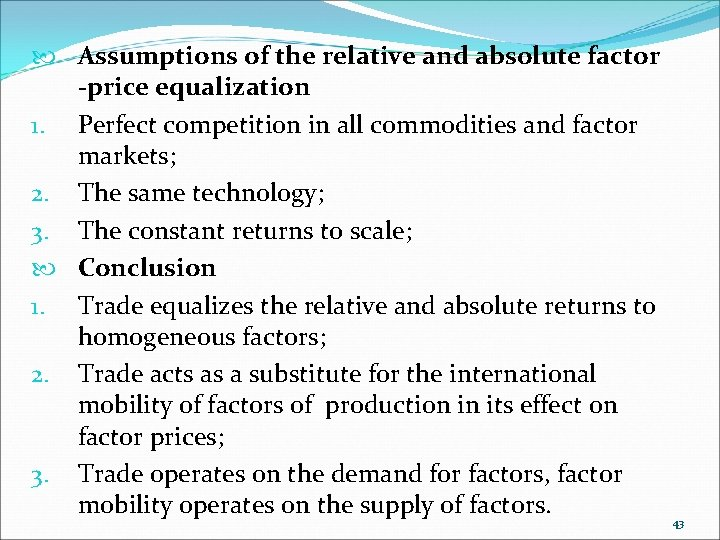Assumptions of the relative and absolute factor -price equalization 1. Perfect competition in all commodities and factor markets; 2. The same technology; 3. The constant returns to scale; Conclusion 1. Trade equalizes the relative and absolute returns to homogeneous factors; 2. Trade acts as a substitute for the international mobility of factors of production in its effect on factor prices; 3. Trade operates on the demand for factors, factor mobility operates on the supply of factors. 43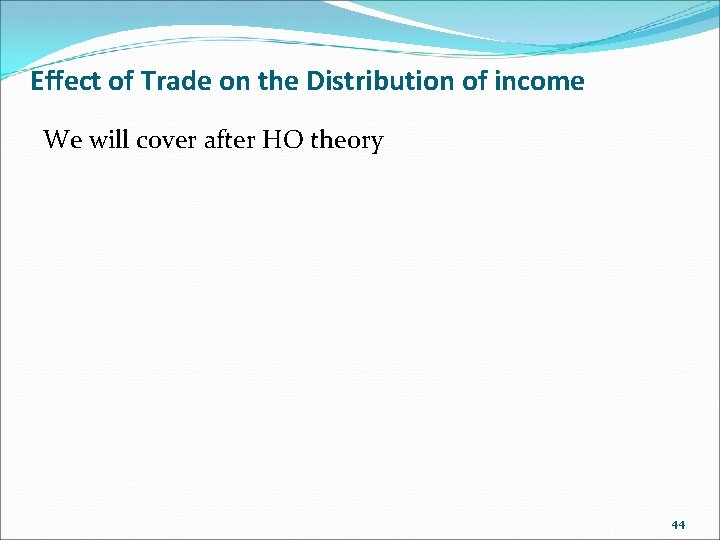Effect of Trade on the Distribution of income We will cover after HO theory 44Factor Price equalization Theorem Empirical Relevance Unreal Assumptions In reality, the equalization of the returns to homogeneous factors is not the case said as H-O -S model in different nations with trade. The reasons as follows: Such as same technology, no transportation cost, free trade, perfect competition and constant returns to scale. 45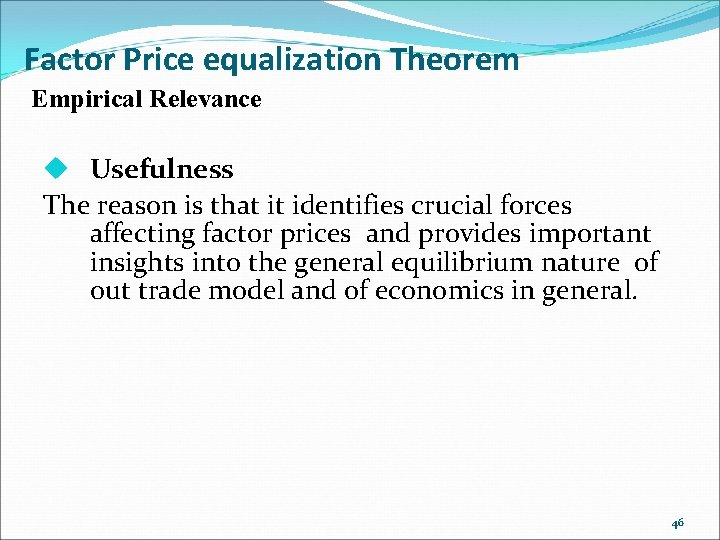Factor Price equalization Theorem Empirical Relevance u Usefulness The reason is that it identifies crucial forces affecting factor prices and provides important insights into the general equilibrium nature of out trade model and of economics in general. 46Factor Price equalization Theorem Empirical Relevance u Shortcoming It doesn’t say that international trade will eliminate or reduce international differences in per capita incomes. It only says that international trade will eliminate or reduce international Differences in the returns to Homogeneous factors. Reason: Per capita incomes depend on other many forces ( The ratio of skilled to unskilled labor and so on). Even if real Wages were to be equalized among nations, their per capita Incomes could be still wider. 47Factor Price equalization Theorem Empirical Relevance Adjustment of H-O-S Model: International trade can reduce the international difference in the returns to homogeneous factors. Reason: even if international trade has operated to reduce the absolute difference in factor returns among nations, many other forces were operating at the same time, preventing any such relationship from becoming clearly evident (e. g. trade restrictions) 485. 6 Empirical Tests of the Heckscher-Ohlin Model n n n Empirical Results-The Leontief Paradox Explanations of the Leontief Paradox Factor-Intensity Reversal 49The Leontief Paradox Leontief's paradox in economics is that the country with the world's highest capital-per worker has a lower capital-labour ratio in exports than in imports. This econometric finding was the result of Professor W W Leontief's attempt to test the HO theory empirically. In 1954, Leontief found that the US(the most capital abundant country in the world by any criteria) exported labour-intensive commodities and imported capital-intensive commodities, in contradiction with Heckscher-Ohlin theory ("H-O theory"). 50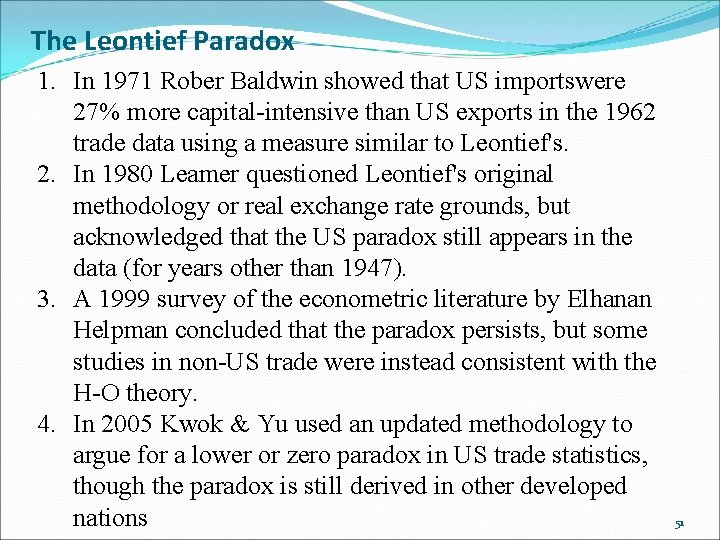The Leontief Paradox 1. In 1971 Rober Baldwin showed that US importswere 27% more capital-intensive than US exports in the 1962 trade data using a measure similar to Leontief's. 2. In 1980 Leamer questioned Leontief's original methodology or real exchange rate grounds, but acknowledged that the US paradox still appears in the data (for years other than 1947). 3. A 1999 survey of the econometric literature by Elhanan Helpman concluded that the paradox persists, but some studies in non-US trade were instead consistent with the H-O theory. 4. In 2005 Kwok & Yu used an updated methodology to argue for a lower or zero paradox in US trade statistics, though the paradox is still derived in other developed nations 51Responses to the Paradox For many economists, Leontief's paradox undermined the validity of the H-O theory, which predicted that trade patterns would be based on countries' comparative advantage in certain factors of production (such as capital and labor). Many economists have dismissed the H-O theory in favor of a more Ricardian model where technological differences determine comparative advantage. These economists argue that the U. S. has an advantage in highly skilled labor more so than capital. This can be seen as viewing "capital" more broadly, to include human capital. Using this definition, the exports of the U. S. are very (human) capital-intensive, and not particularly intensive in (unskilled) labor. 52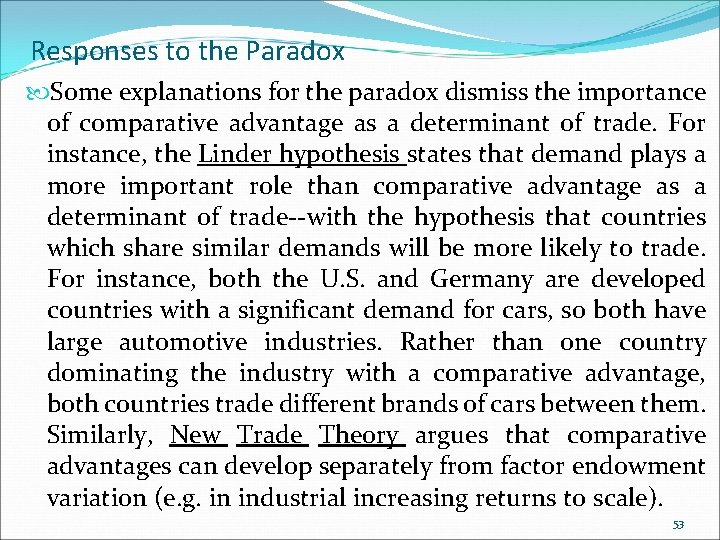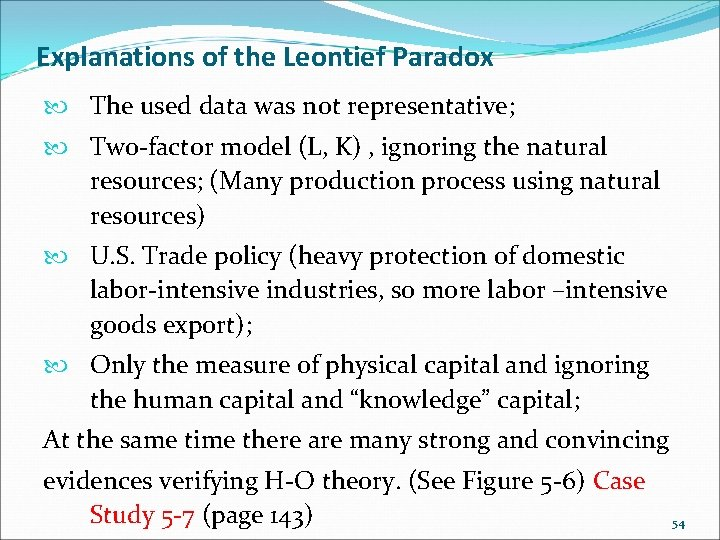Explanations of the Leontief Paradox The used data was not representative; Two-factor model (L, K) , ignoring the natural resources; (Many production process using natural resources) U. S. Trade policy (heavy protection of domestic labor-intensive industries, so more labor –intensive goods export); Only the measure of physical capital and ignoring the human capital and “knowledge” capital; At the same time there are many strong and convincing evidences verifying H-O theory. (See Figure 5 -6) Case Study 5 -7 (page 143) 54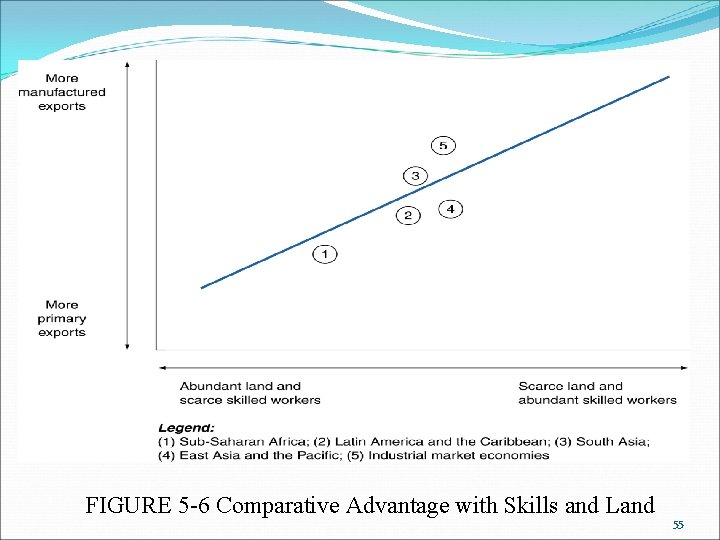FIGURE 5 -6 Comparative Advantage with Skills and Land 55Effect of International Trade on distribution of Income International trade on the effect of relative factor prices within each nation u Trade increases the price of the nation’s abundant and cheap factor and reduces the price of its scarce and expensive factor. eg W rises and r falls in Nation 1 while w falls and r rises in Nation 2. International trade on the effect of income within each nation u The real income of labor and the real income of owners of capital move in the same direction as the movement in factor prices. eg Trade causes the real income of labor to rise and the real income of owners of capital to fall in Nation 1 while in Nation 2 the situation is the opposition. (Stolper-Samuelson Theorem) 56Effect of International Trade on distribution of Income Stolper-Samuelson Theorem: The theorem postulates that an increase in the relative price of a commodity raises the return or earnings of the factor used intensively in the production of the commodity. Application u Developed countries are the relatively capital abundant Factor, international trade tends to reduce the real income of labor and increase the real income of owners of capital. This is why labor union generally favor trade restrictions. ( Case study 5 -5 page 139) 57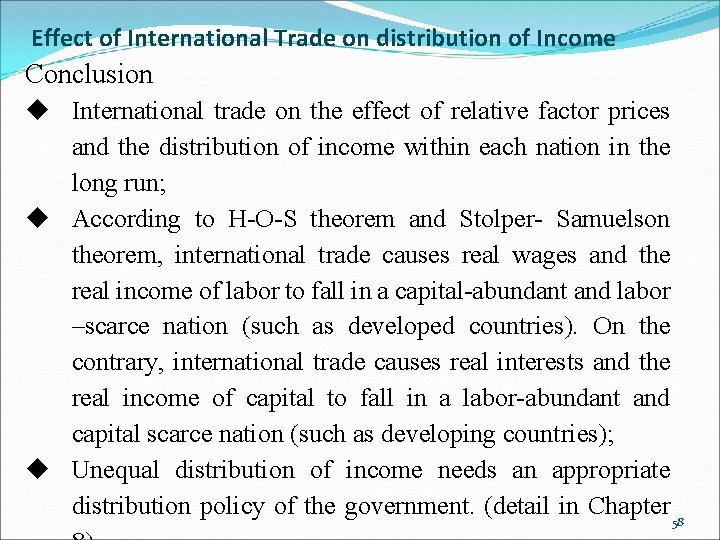Effect of International Trade on distribution of Income Conclusion u International trade on the effect of relative factor prices and the distribution of income within each nation in the long run; u According to H-O-S theorem and Stolper- Samuelson theorem, international trade causes real wages and the real income of labor to fall in a capital-abundant and labor –scarce nation (such as developed countries). On the contrary, international trade causes real interests and the real income of capital to fall in a labor-abundant and capital scarce nation (such as developing countries); u Unequal distribution of income needs an appropriate distribution policy of the government. (detail in Chapter 58The Specific-Factors Model Conclusion In the short run when some factors my be immobile Or specific to some industry or sector. In this case, it Postulates that trade will have an ambiguous effect on the nation’s mobile factors : It will benefit the immobile factors that are specific to the nation’s export commodities or sectors, and harm the immobile factors that are specific to the nation’s import-competing commodities or sectors. 59The Specific-Factors Model Conclusion In the long run when all input are mobile among all Industries of a nation, the H-O model postulates that the opening of trade will lead to an increase in the real income or return of the inputs used intensively in the nation’s export sectors and to a reduction in the real income or return of the inputs used intensively in the production of the nation’s import-competing sectors. 60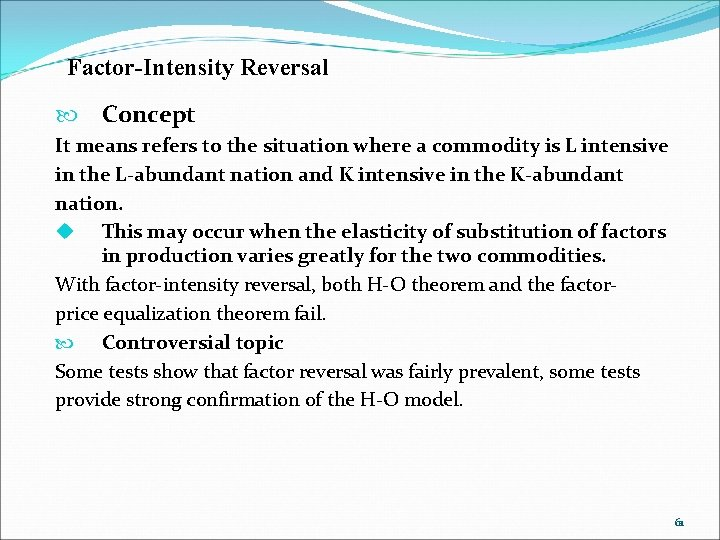Factor-Intensity Reversal Concept It means refers to the situation where a commodity is L intensive in the L-abundant nation and K intensive in the K-abundant nation. u This may occur when the elasticity of substitution of factors in production varies greatly for the two commodities. With factor-intensity reversal, both H-O theorem and the factorprice equalization theorem fail. Controversial topic Some tests show that factor reversal was fairly prevalent, some tests provide strong confirmation of the H-O model. 61